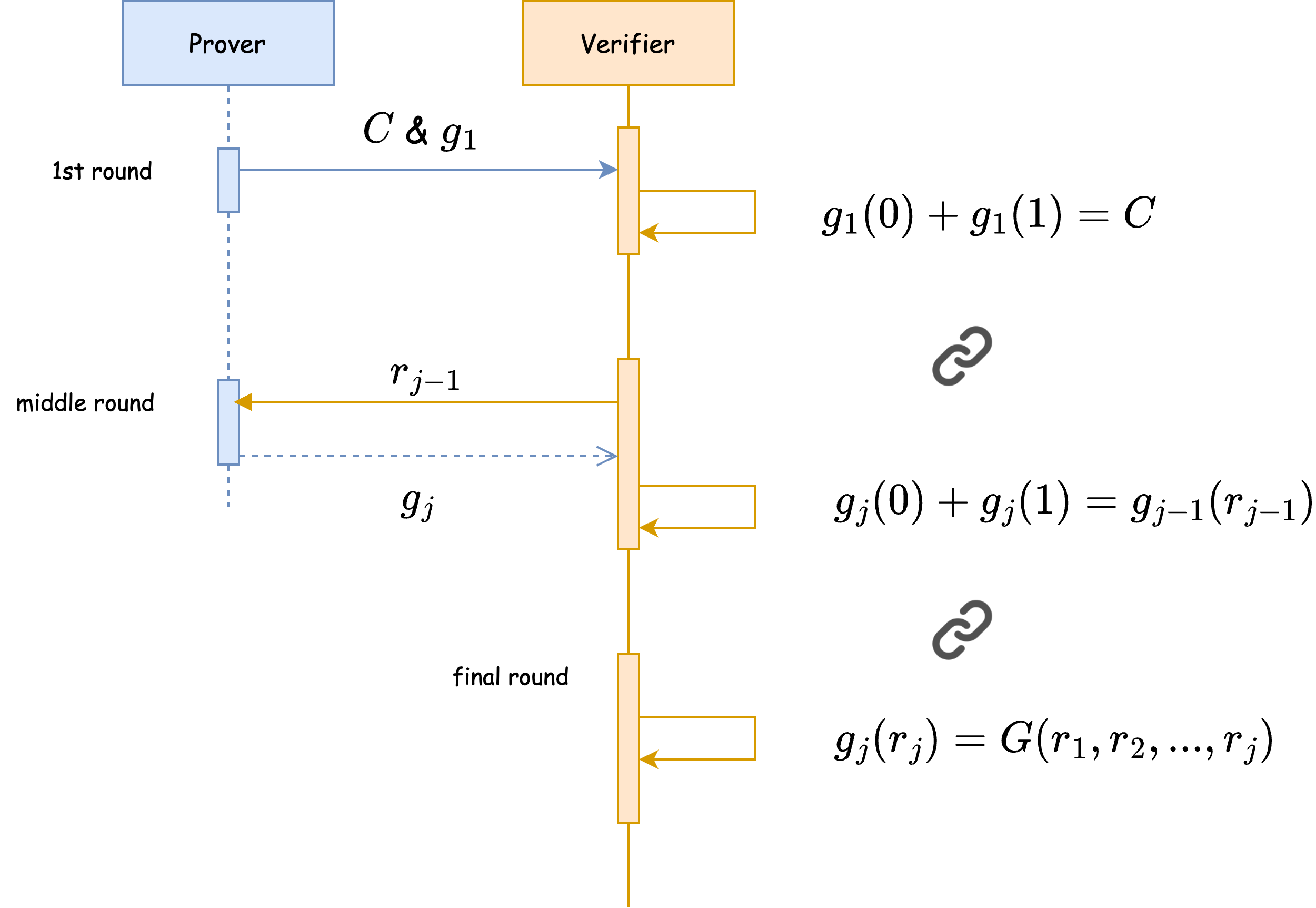# Sum Check Protocol - part 1: Overview

## Introduction

This is the part 1 of a blog post series about sum check protocol. The knowledge is mainly derived from the book Proofs, Arguments, and Zero-Knowledge by Justin Thaler and its study group.

The emphasis of this series is on the use case of triangle counting and benchmarks with different implementations at low level. So it could be served as another dimensions to understanding the protocol with counting triangles as the scene.

One of the goals is to iterate it into practical zero-knowledge use cases with implementations from scratch, such as algorithms for multilinear extension and univariate polynomial generation etc. I found working with these low level implementations is beneficial to digest the knowledge especially relating to maths, which seems very important when it comes to optimizing benchmarks, debugging or simplifying explanation for real world applications.

I hope it can provide a lower entry point for anyone interested in learning the basis of zero-knowledge techniques with various levels of math maturity, while it could become a long term side project for me to make continuous experiments or a framework easy for anyone who are intrigued to challenge my implementations for better benchmark performance.

In this article, it focuses on providing an overview to the sum check protocol with a diagram illustrating the workflow at high level, which aims to ease growing a mental model around the protocol in addition to 4.1 section in the book.

Part 2 will introduce how triangle counting can be done using adjacency matrix as a graph with diagrams for step by step illustration, and how to represent it into a polynomial, which then can be feed into the sum check protocol.

Part 3 will combine the concepts introduced from the prior parts, and brief how it structures the code for different implementations and makes it easier for benchmarking new implementations.

There are already a couple of articles with implementations, written by the community for the same topic. The accompany implementations of this blog post series got inspirations from both of them, and extend the implementation from 0xsage. I highly recommend checking them out as well.

## Benefit

In short, sum check protocol aims to save verifier from re-calculating from scratch the truth claimed by prover.

In other words, the protocol is to guarantee prover tells the truth of a calculation result while the verifier only needs to spend significant less calculation resource to verify the truth.

## Protocol workflow

In the implementation, there are two structs representing the roles of prover and verifier as illustrated in the diagram. Here is a test demonstrating the high level interactions between them.

Note that the test case involves triangle counting, which will be explained in Triangle counting. For now, the code references serve as a warm up for you to understand the protocol in different dimensions, which are the diagram, code and description in the post.### First round

• Prover
1. Calculates the result as $C$ for the sumcheck function $C:=\sum _{{b}_{1}\in \left\{0,1\right\}}\sum _{{b}_{2}\in \left\{0,1\right\}}\dots \sum _{{b}_{v}\in \left\{0,1\right\}}g\left({b}_{1},\dots ,{b}_{v}\right)$.
$C$ is the truth claimed by the prover. For the triangle counting, the $C$ can be calculated from anyways, either in hypercube sum or matrix multiplication.
2. According to the equation, ${g}_{j}\left({X}_{j}\right)=\sum _{\left({x}_{j+1},\dots ,{x}_{v}\right)\in \left\{0,1{\right\}}^{v-j}}g\left({r}_{1},\dots ,{r}_{j-1},{X}_{j},{x}_{j+1},\dots ,{x}_{v}\right)$, generates the univariate polynomial ${g}_{1}\left({X}_{1}\right)$ from the multivariate sumcheck polynomial for the fixed variable ${X}_{1}$ with index based on the current round of the protocol interactions. Note that it is in the 1st round, so is ${g}_{1}\left({X}_{1}\right)$.
3. Provides $C$ for verifier to construct and ${g}_{1}\left({X}_{1}\right)$ to check.
• Verifier
4. Checks ${g}_{1}\left(0\right)+{g}_{1}\left(1\right)=C$

### Middle rounds

• Verifier
1. Advances the current round flag and generate random number ${r}_{j-1}$.
• Prover
2. Generates an univariate polynomial ${g}_{j}\left({X}_{j}\right)$ with the set of $\left({r}_{1},...,{r}_{j-1}\right)$ and send it to verifier.
• Verifier
3. Check ${g}_{j}\left(0\right)+{g}_{j}\left(1\right)={g}_{j-1}\left({r}_{j-1}\right)$

### Final round

For verifier, after done checking ${g}_{j}\left(0\right)+{g}_{j}\left(1\right)={g}_{j-1}\left({r}_{j-1}\right)$ in the previous round, it checks ${g}_{j}\left(0\right)+{g}_{j}\left(1\right)=G\left({r}_{1},{r}_{2},...,{r}_{j}\right)$, where $G$ is the multilinear extension form of sumcheck polynomial $\sum _{{b}_{1}\in \left\{0,1\right\}}\sum _{{b}_{2}\in \left\{0,1\right\}}\dots \sum _{{b}_{j}\in \left\{0,1\right\}}g\left({b}_{1},\dots ,{b}_{j}\right)$.

Note the reason to have "multilinear extension" transformation for the sum check polynomial is because it can evaluate random numbers other than $\left\{0,1\right\}$ for each variable in the protocol.

### Soundness

In other words, how does the protocol guarantee the prover tells the truth while having the performance gain in the verifier time?

Intuitively, as the workflow illustrates, the verifier does verifications that chain up between the facts represented by $C$, ${g}_{j}\left({X}_{j}\right)$ and $G\left({r}_{1},{r}_{2},...,{r}_{j}\right)$. To fake the soundness, the prover has to fake all the information provided to verifier. This is extremely unlikely to succeed when the random numbers belongs to a finite field where the size is much greater than the variable number.

As the soundness guarantees , the verifier only needs to evaluate low degree ${g}_{j}\left({x}_{j}\right)$ and evaluation over one point $G\left({r}_{1},{r}_{2},...,{r}_{j}\right)$, the combination of both is still much faster than calculating from scratch.

For the mathematical proof of the soundness, please refer to the 4.1 in the book.

## Use case

Sum check protocol is applicable to use cases in which the results requires summing up over all the possible domains. Triangle counting via adjacency graph requires thorough walk through all different paths, thus is an applicable use case for the protocol.

In the part 2, it will try to introduce the triangle counting problem in order to lay down a fundamental mental model for context of sum check protocol.